# PDF Cylindrical Quantum Dots, Spin Qubits, and Decoherence - ERNET

N/A
N/A
Protected

Share "PDF Cylindrical Quantum Dots, Spin Qubits, and Decoherence - ERNET"

Copied!
126
0
0

Full text

We show that this can be achieved by manipulating only the strength of the external magnetic field. We also see how the decoherence process depends on the nature of the interaction included in the model. 2.19) as a function of a1 21 2.3 Variation of the electron probability density with magnetic field strength 22 2.4 Variation of radial energy levels with magnetic field strength for.

## Quantum States

Since the position basis is not special as far as the LVS of a quantum system is concerned, similar superpositions also exist in other basis representations of the state vector. Therefore, if the quantum state is prepared in a linear superposition of two energy eigenstates, for example |1i and |2i, the time evolution of the state can be written as.

## Two-electron System

Because the Schrodinger equation is linear in the wave function, the state of a two-electron system can be represented by a linear combination of the form. For the two electron states, this would require definite but opposite symmetries for the orbitals and spins of the state lattice.

## Quantum Information Technology

Feynman who pointed out that simulating quantum mechanics on a classical computer can be computationally expensive. On the other hand, Benioff showed how a classical Turing machine could be simulated by the reversible unitary evolution of a quantum process .

Semiconductor Quantum Dots

## Loss-DiVincenzo Proposal

One of the proposals was to use vertically tunneled quantum dots in the presence of external electric and magnetic fields . An experimental demonstration of the coherent control over such an encoded qubit is also reported in the literature .

## Decoherence

Since the microscopic state of the environment is inaccessible to us, the phenomenon of decoherence is irreversible. Decoherence is an extremely fast process for macroscopic objects since the number of degrees of freedom of the environment interacting with such objects is very high.

## Outline of the Thesis

We show how the states and energy levels depend on the strength of the magnetic field for different quantum numbers. In Chapter 3 we study the confinement of a single electron in the hydrogen impurity potential in the presence of a magnetic field.

## Theory

Some of such explorations using cylindrical quantum dot structure in the presence of a magnetic field are listed in the references. The value of quantum number n corresponds to the number of nodes in the radial part of the wave function.

## Results and Discussion

Here, ωc is the cyclotron frequency and is directly proportional to the magnetic field strength. In the case of a large diameter dot, the merging of energy levels occurs immediately with an increase in the strength of the magnetic field.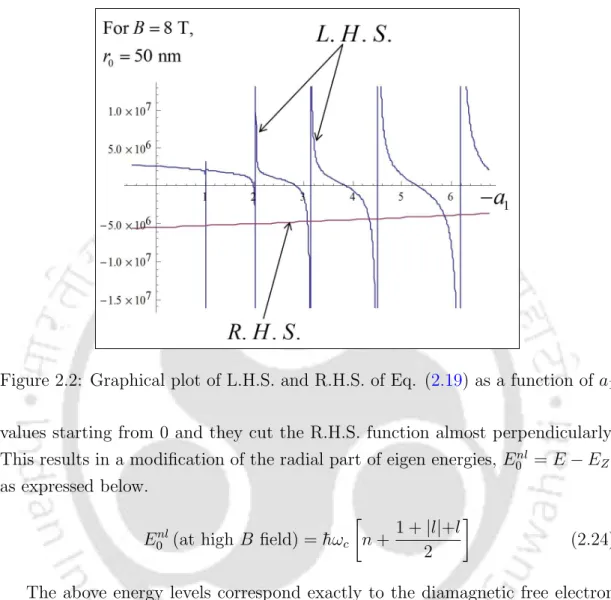Figure 2.2: Graphical plot of L.H.S. and R.H.S. of Eq. (2.19) as a function of a 1

## Conclusion

In some of the cases, the system can be treated approximately as a two-dimensional problem. A hydrogen impurity will lead to a logarithmic potential in a strictly two-dimensional world due to Gauss's law in two dimensions.

## Theory and Numerical Procedure

### Matrix Method

Here rmax is a large enough value of r that the wave function can for all practical purposes be taken to be zero. The matrix method with higher order accuracy in approximating the second derivative term is also considered in our research. Thus, we look for a method which can include a non-uniform grid with more samples in the r= 0 region.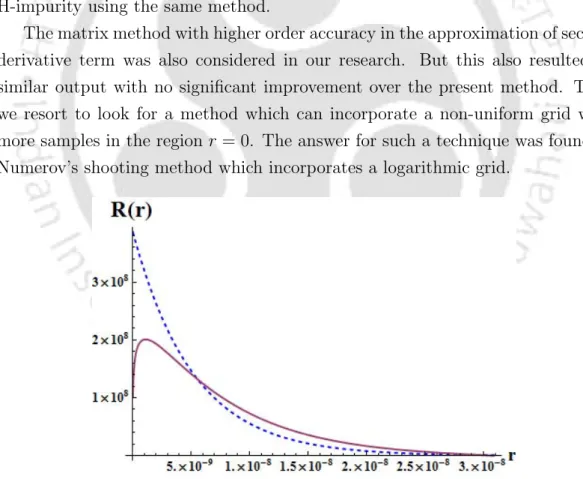Figure 3.1: Comparison of analytical (dashed) and numerical (continuous) solu- solu-tion based on matrix method

### Numerov’s Shooting Method

It should also be noted that the greater the number of nodes in a wave function, the greater the corresponding eigenenergy will be. The number of nodes in the function fL(r) is counted by tracking the number of times the function changes sign until it reaches the intermediate point rc that we have identified. It is noticed that the current solution corresponds worse to the exact solution compared to that in the matrix method from the previous subsection.

### Logarithmic Grid

Figure 3.5 shows the radial plot of the ground state wave function for different external magnetic flux densities. This is because the rate of increase in the energy of a state depends on the value of l. The variation of the excited state wave functions with respect to the magnetic field is shown in Fig.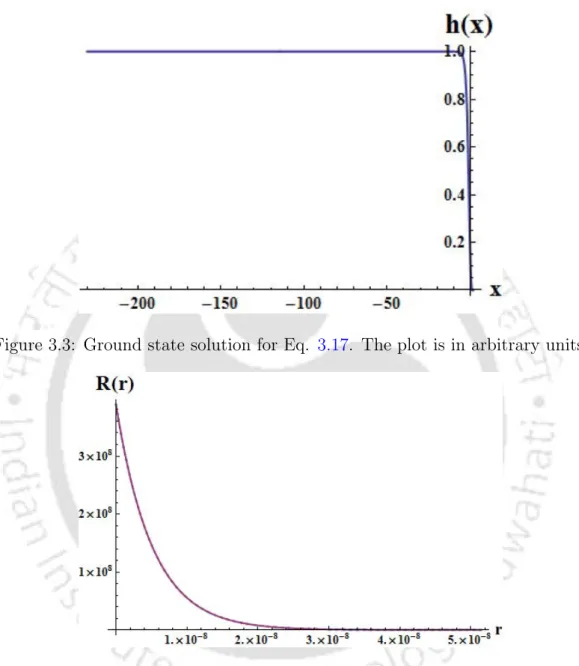Figure 3.3: Ground state solution for Eq. 3.17. The plot is in arbitrary units.

## Introduction

For a point with a very small height-to-diameter ratio, we can safely assume that electrons are confined to the ground state subband in the z direction and thus the problem reduces to the case of a two-dimensional circular disk. In the present work, we use linear variational analysis where the trial wavefunction is constructed from single-electron harmonic oscillator wavefunctions.

## Theory and Procedure

For each case, the linear analysis of variation reduces to solving an eigenvalue problem of the type. Now the matrix [Z] is diagonal due to the orthonormality of the orbital part of the basic wave functions. Similarly, many of the elements in [W] are zeros due to the othogonality condition when m1 6= m3 or m2 6= m4.

## Results and Discussion

The energy spectrum obtained for different quantum numbers is plotted as a function of the external magnetic field strength in Figure. Similarly, singlet-triplet crossing is observed for the ground state, with the increase of external magnetic field. We also mapped the radial electron densities and pair correlation functions for different quantum numbers (M, s) and magnetic field strengths.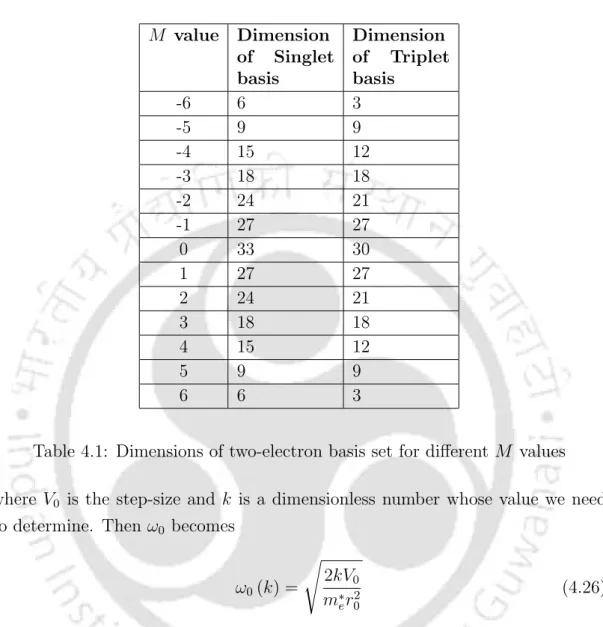Table 4.1: Dimensions of two-electron basis set for different M values where V 0 is the step-size and k is a dimensionless number whose value we need to determine

## Conclusion

Due to the inherent antisymmetry in the orbital wave function of the triplet states, fpc(0) = 0, because no two electrons in such states can be in the same place. This can be considered a very good approximation, as the changes in effective mass and permeability are known to be very small with respect to material composition. In the case of self-assembled quantum dots, experimental results indicate a violation of the general Kohn theorem, which indicates discontinuities in the confinement potential [124; 125].

## Theory

### Heitler-London Approximation

If ϕL(~r) is the ground state of the left QD and ϕR(~r) is the ground state of the right QD, the orbital part of the two-electron wave function is written as In the above expression, the energy of one electron for the ground state with one point, Vr(r) =V(r)−VL(r) and Vl(r) =V(r)−VR(r) are the residual potentials ;. Shifting the ground state orbital of one particle left and right by the transformation (x, y) → (x± a, y) will also change the scale.

### Weinbaum Approximation

C} is a column matrix corresponding to the linear variation parameters to be determined, and E is the unknown energy eigenvalue. G] is the overlap matrix written in the same basis and its expression is given by We expect that introducing doubly occupied states into the analysis improves the value of the exchange energy.

## Results and Discussion

The separation between dots 2a= 1.4r0 in the H-L method (dotted) and the Weinbaum method (solid) is taken into account here. Here, the point-to-point separation of 2a = 1.6rB is considered for the H-L method (dotted) and the Weinbaum method (solid). It can also be seen that the magnetic field at which the singlet-triplet crossing occurs is also a function of the dot separation.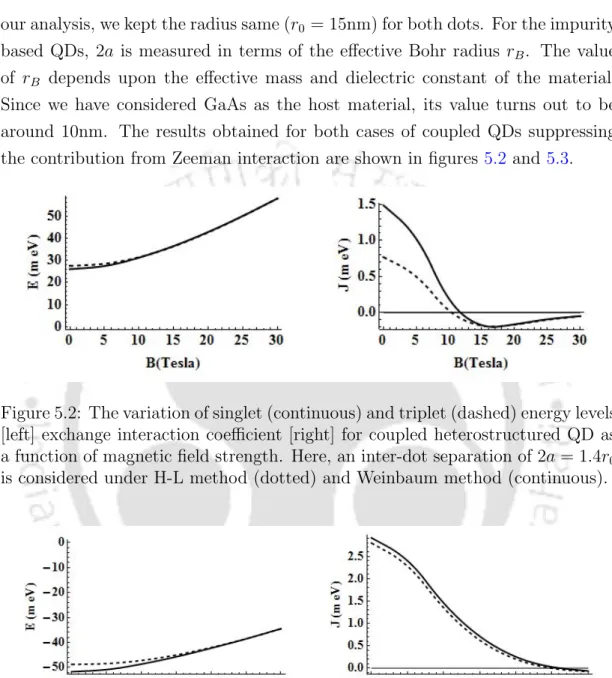Figure 5.2: The variation of singlet (continuous) and triplet (dashed) energy levels [left] exchange interaction coefficient [right] for coupled heterostructured QD as a function of magnetic field strength

## Application in Quantum Computation

But for smaller QD radius, the singlet-triplet transition occurs at larger values ​​of external magnetic field. This can be done by operating the system at a magnetic field strength, Bc, where singlet and triplet states have the same energy, then switching the field to a non-zero exchange interaction energy, and then allowing the system to evolve for a sufficient time, τg. The different graphs correspond to exchange interaction between the lowest singlet state and the three non-degenerate triplet states, viz.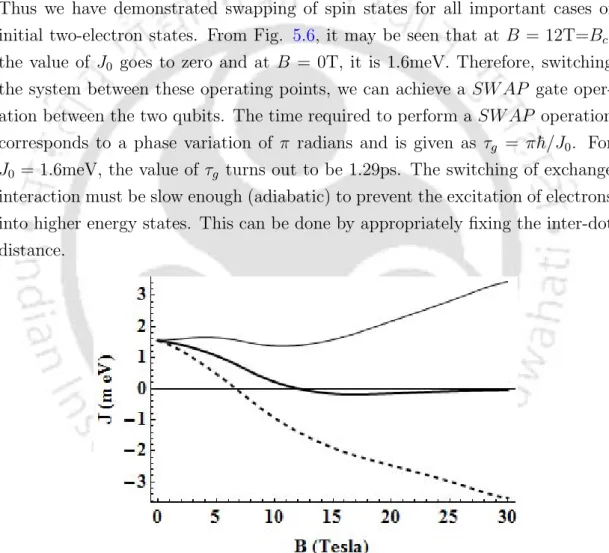Figure 5.6: The variation of exchange interaction coefficient for coupled het- het-erostructured QDs as a function of magnetic field strength

## Conclusion

In most applications we are only interested in the quantum state of the electrons and not in the state of the environment around them. Here, the first term represents the Hamiltonian of the central spin in the absence of any interaction with the spin bath. The second term represents the contribution to the Hamiltonian due to the interaction with the nuclear spin in the spin bath, where Jk indicates the strength of hyperfine interaction between the central electron spin and kth nuclear spin of the bath.

## Theory

Where a and b are complex probability amplitudes of electron spins found in up (|0i) and down (|1i) states, and cn is the probability amplitude of the product state (|ni) of the nuclear spin pool containing N nuclear spins. But the square of the standard deviation of is equal to the sum of the squares of the individual standard deviations as given in Eq. In the following, we consider three distinct quantum dot geometries and analyze how Λ2 depends on the dimension of the nuclear spin bath.

## Results and Discussion

This is due to quantum confinement effects, as can be directly verified from the expressions for J(~r) in each case. The solid-thick, dashed, dotted, and continuous-thin curves correspond to u = 0, u = 0.2, u = 1, and u = 5, respectively. you notice complete decoherence, since the initial state is not even an eigenstate. system–Hamiltonian, nor the interaction term. This is because our current initial state is the eigenstate of the interaction—the Hamiltonian, which is the only term governing the evolution of the system when u = 0.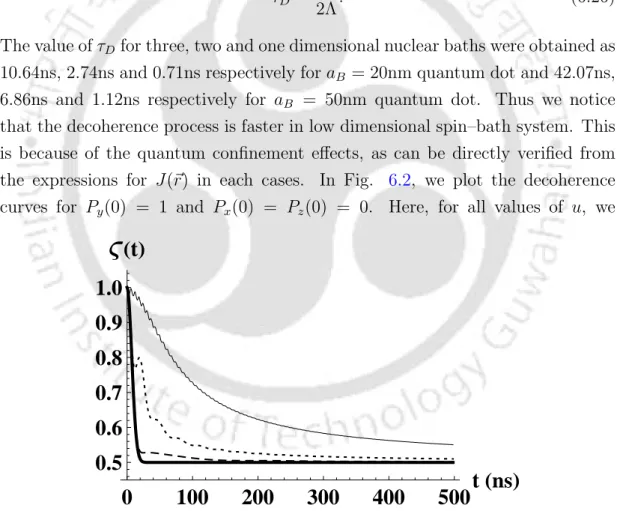Figure 6.2: The time development of decoherence when |ψ S (0)i = √ 1

## Conclusion

The main physical mechanisms that limit the coherence time of spin qubits are spin-orbit interactions and interactions with nuclear spins. As a final problem in this work, we study the decoherence of two electron spin qubits in a coupled quantum dot due to their interaction with the surrounding nuclear spin bath. We use an extended version of the simplified model discussed in the previous chapter on single-electron decoherence.

## Theory

### Characteristics of two-qubit density matrices

From the two-qubit density matrix one can evaluate quantitative measures such as ς(t) =Trρ2AB(t) and simultaneity. If the elements of the matrix are functions of time, the polarization components as well as the quantitative measures. The plot of ς(t) with respect to time can tell us how the quantum coherence of the two-qubit state is lost as a function of time.

### Continuum Limit

Therefore, if we assume that all individual nuclei in the nuclear bath possess the same statistics, then the qubit-bath interaction energies, A = R. In such cases, the qubit-bath interaction energies are completely correlated, that is, we can define A = B. Now we can overcome the summation in Eq. 7.7with an integration over A and B, the qubit-bath interaction energy variables for electron A and electron B. The limits of integration range from min = −R. Thus, the polarization components of the two-qubit density matrix at time t. 7.17) where 2Nf(A, B, t)dAdB is the number of bath product states with the first qubit bath interaction energy between A and A+dA and the second qubit bath interaction energy between B and B+dB.

## Results and Discussion

When Jex = 0, the qubit-bath interaction energies A and B can be considered to be independent of each other. Since this is a linear combination of two triplet states, we have only one variable of the qubit-bath interaction energy. This is because the value of Λ, the standard deviation of the qubit-bath interaction energy distribution function, depends on the spread of the wave function.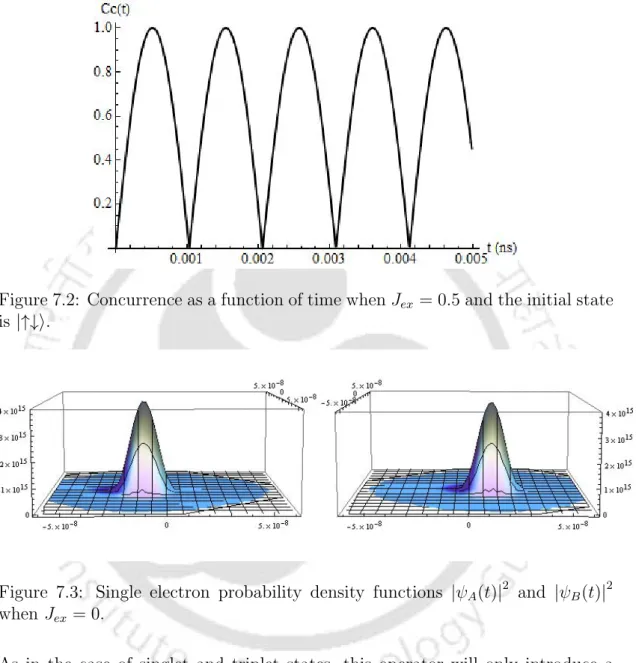Figure 7.2: Concurrence as a function of time when J ex = 0.5 and the initial state is |↑↓i.

## Conclusion

We also plotted the variation of energy levels with the flux density of the applied magnetic field. In the last part of the thesis, we studied the decoherence of spin qubits in quantum dots due to their hyperfine interaction with the surrounding nuclear bath environment. The decoherence process therefore depends on the initial state of the system as well as the relative strengths of different terms in the Hamiltonian.

Figure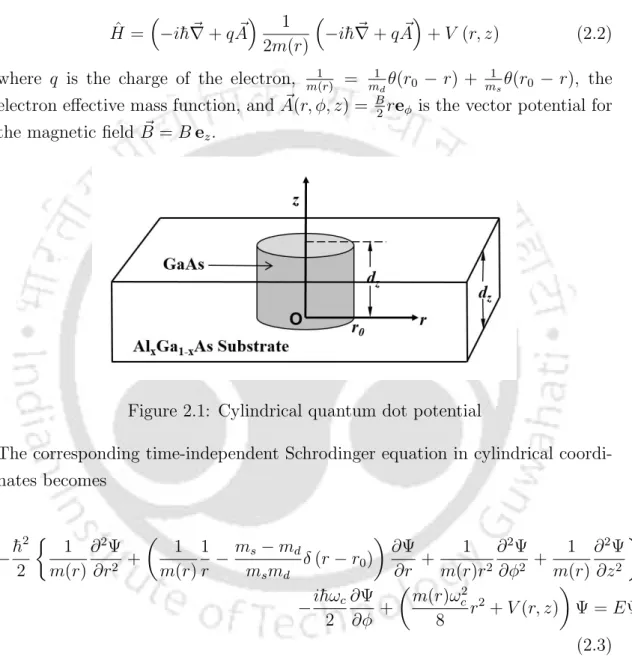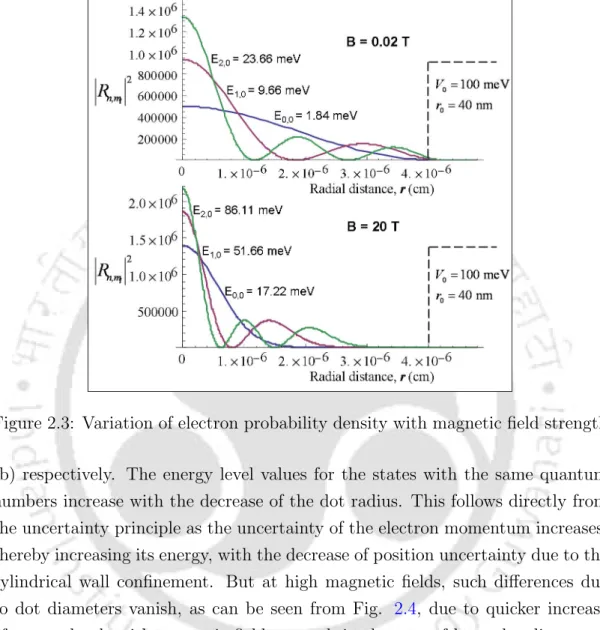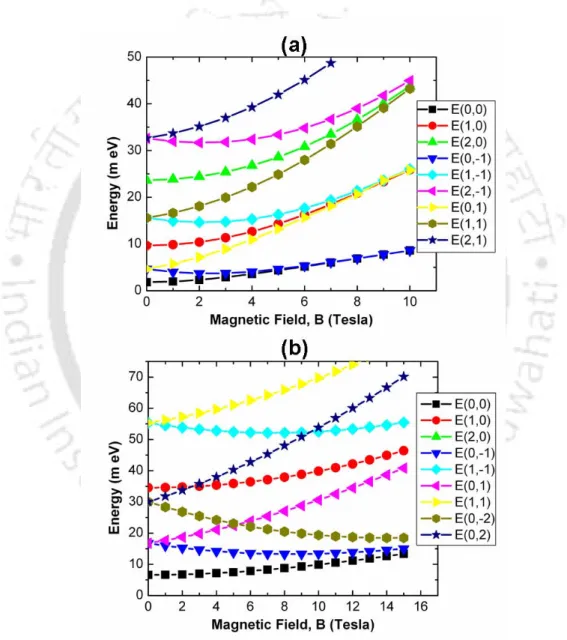+7

References

Related documents

Irrespective of the spin topologies of the diﬀerent phenyl rings, the non-selective excitation of homonuclear highest quantum coherence results in ﬁltering of sub spectra for each

1-2 Research problem: One of the problems that the athlete faces, which poses a threat to the athlete’s future, is the injury to the anterior cruciate ligament, and the suffering of

Inferences: It is identified that there are certain individuals or groups who are buzzing about any financial plan, concepts, package policy, schemes or in any form will influence the

NOMENCLATURE POCP Parabolic Optimal Control Problem PBCP Parabolic Boundary Control Problem LDMF Lower Dimensional Manifold Rd d-dimensional Euclidean space Ω Bounded convex domain

In chapter 2, we studied the bistable behavior shown by the intracavity optical field and mechanical mirror motion in a hybrid optomechanical system consisting of a driven

The primary texts are The Tiger’s Daughter 1971, Wife 1975, Jasmine 1989, Desirable Daughters 2002, and The Tree Bride 2004.The Holder of the World 1993, and Leave It to Me 1997 have

Thesis Title: Controlled Growth of Organo-Metal Halide Perovskite Quantum Dots and Two dimensional Nanosheets for Blue Light Emission and Photodetection Name of Thesis Supervisors :

Keywords: Cardiovascular diseases, COVID‑19, diabetes, healthcare services, hypertension, noncommunicable diseases NCDs IntroductIon A novel coronavirus disease 2019 COVID‑19, caused

Once patients are initiated on basal insulin therapy, at some point, mere increasing basal insulin dose will not be enough to maintain desired glycaemic control, eventually requiring